Succeed with maths – Part 1

Start this free course now. Just create an account and sign in. Enrol and complete the course for a free statement of participation or digital badge if available.

Free course

1.2 Money

You will probably see percentages used most often in your everyday life when money is involved: discounts in shops, for example, or how much tax you have to pay on your income. All the same principles apply when dealing with percentages associated with money, so use what you have learned so far in the following activities.

Activity 3 Where does the money go?

Timing: Allow approximately 10 minutes

Let’s suppose somebody’s gross monthly income (that is, the income before taxes) is £1250. From this money, £315 a month goes on paying housing costs and £135 is saved. Work out the percentage (to 1 decimal place) of the gross monthly income that goes towards:

• a.housing costs
• b.savings.

Remember to click on the ‘Reveal comment’ button if you need a hint.

Comment

Think of these as fractions first before trying to find the percentage.

• a.Write the numbers as a fraction first: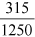.

Now convert to a percentage by multiplying by 100 %.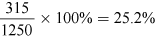(to 1 decimal place)

So 25.2% of the gross income is spent on housing costs.

• b.Using the same method as in part (a), the percentage towards savings =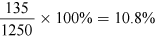(to 1 decimal place).

Of course, if you had started with the net pay (after tax has been deducted), these percentages would have been larger because you would be comparing the savings and housing costs to a lower figure.

Now let’s look at a different type of saving: putting something aside for retirement.

Activity 4 Saving for retirement

Timing: Allow approximately 10 minutes

Many people think that saving for retirement is important. How much somebody needs to save or can afford to save will depend upon many factors, including when they want to retire and when they start saving for retirement.

One way to get an estimate of what percentage of gross salary (before tax) individuals should be putting towards a pension is to base it upon their age when they start saving. Fortunately, there is a quick way to do this. The percentage to save is found by halving the age of the individual at the time they plan to start saving. So if somebody planned to starting saving at 50, they would need to save 25 per cent of their gross salary. (Obviously these are just estimates – you would need to take advice from a financial expert for your own situation!)

Use this information to calculate the amounts that the following people should be putting into a pension fund:

• a.A 20-year-old with a gross salary of £15 000.
• b.a 35-year-old with a gross salary of £25 000.
• c.a 45-year-old with a gross salary of £43 000.

• a.Percentage to save = half the age = 10%

Converting the percentage to a decimal for the calculation:

10% = 10 ÷ 100 = 0.1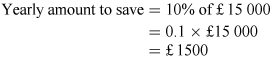• b.Percentage to save = half the age = 35 ÷ 2 = 17.5%

Converting the percentage to a decimal for the calculation:

17.5% = 17.5 ÷ 100 = 0.175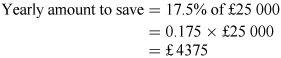• c.Percentage to save = half the age = 45 ÷2 = 22.5%

Converting the percentage to a decimal for the calculation:

22.5% = 22.5 ÷ 100 = 0.225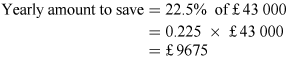You could have also calculated all these answers by converting the percentages to fractions.

Hopefully you are now feeling even more confident about working with percentages. Now that you’ve looked at the basics, you are going to build on this confidence in the next section and move on to another stage. Keep in mind that percentages are a specific kind of fraction, where the denominator is always 100. First you’re going to look at whether the order that you use a percentage increase or decrease matters.